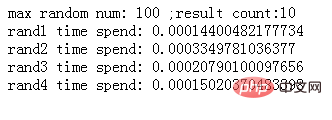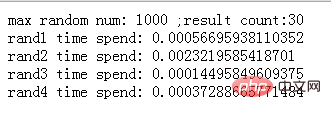```<?php
define('RANDOM_MAX', 100);
define('COUNT', 10);

echo 'max random num: '.RANDOM_MAX, ' ;result count:'.COUNT, '<br/>';

invoke_entry('rand1');
invoke_entry('rand2');
invoke_entry('rand3');
invoke_entry('rand4');

function invoke_entry(\$func_name) {
\$time = new time();
\$time->time_start();
call_user_func(\$func_name);
echo \$func_name.' time spend: ', \$time->time_spend();
echo '<br/>';
}
function rand1() {
\$numbers = range (1, RANDOM_MAX);
shuffle(\$numbers);  //随机打乱数组
\$result = array_slice(\$numbers, 1, COUNT);
return \$result;
}
function rand2() {
\$result = array();
while(count(\$result)< COUNT) {
\$result[] = mt_rand(1, RANDOM_MAX); //mt_rand()是比rand()更好更快的随机函数
\$result = array_unique(\$result); //删除数组中重复的元素
}
return \$result;
}
function rand3() {
\$result = array();
while(count(\$result) < COUNT) {
\$_tmp = mt_rand(1, RANDOM_MAX);
if(!in_array(\$_tmp, \$result)) { //当数组中不存在相同的元素时，才允许插入
\$result[] = \$_tmp;
}
}
return \$result;
}
function rand4() {
\$result = array();
while (count(\$result) < COUNT) {
\$result[] = mt_rand(1, RANDOM_MAX);
\$result = array_flip(array_flip(\$result));  //array_flip将数组的key和value交换
}
return \$result;
}
class time {
private \$_start;

public function time_start() {
\$this->_start = \$this->microtime_float();
}
public function time_spend() {
return \$this->microtime_float() - \$this->_start;
}
private function microtime_float() {
list(\$usec, \$sec) = explode(" ", microtime());
return ((float)\$usec + (float)\$sec);
}
}

?>```php怎么实现数组随机且不重复